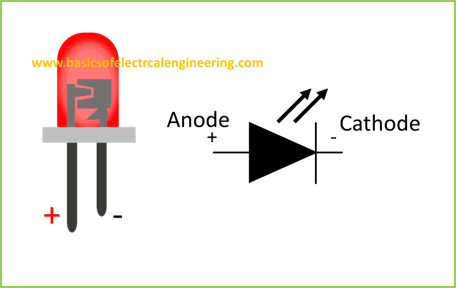# Basics of Electrical Engineering

Learn the basics of Electrical Engineering.

In this article, you'll learn the basics of most commonly used electrical components.
Component Detail
Resistor It controls the amount of current flowing through circuits.
Breadboard A prototyping board on which components are connected on the temporal basis.
LED The electronic component which emits electrical light.
Capacitor It stores electrical charge
Inductor The electronic component which stores energy in the magnetic field.
Connecting wires Used to join components together.
Multimeter A device which measures electrical quantities.
Oscilloscope A device which measures ac signals and plots them on a screen.
Function generator A device which generates different signals.
Let's start our learning.

### Resistor

The resistor is the most commonly used component in any circuit. Perhaps 100% of circuits use it. Current and resistance are two basic properties of electrical engineering. The amount of current flowing through any circuit is inversely related to the resistance.
Consider a circuit with 10 V battery and a 10-ohm resistance. The amount of current in this circuit as per Ohm's law is 1 A.
Practically your circuit application requires different amounts of current. Depending on your application you might need 0.5 ampers or 0.1 mA or 0.2 amperes. You can achieve the required current by changing the value of resistance. For finding the exact amount of current you need to apply the Ohm's law formula which is R = V/I. Let's check out these examples which illustrate the entire way.
Fortunately, there is an online calculation tool which helps you to do it automatically. However, you should know the formula and the way to apply it.

The breadboard is a prototyping board which comes in various models and shapes. You can use a breadboard to connect components on a temporary basis. A common model of the breadboard is shown below. The columns and rows are interconnected in some patterns.
The image is self-explanatory. However, you can learn the method of making circuits here.

### LED

LED stands for light emitting diode. It is two terminal device, one being the anode and another one is the cathode. You can easily differentiate between anode and cathode of led by noting the longer leg.### Capacitor

It is an electronic component which stores charge.

### Inductor

An inductor is a coil of wire which stores energy in its magnetic field.

### Connecting wires

These are small-sized conductors which are used to join the components.

### Multimeters

Multimeters are the measuring devices which contain two probes. These probes are connected to the circuits and are used to measure voltage, current, and resistance.

### Oscilloscope

Digital oscilloscope takes the input and displays the signal on the screen.

### Function generator

A device which generates the ac signals for various functions such as sine, square, triangle.
Let's wrap up our discussion with this infographic: